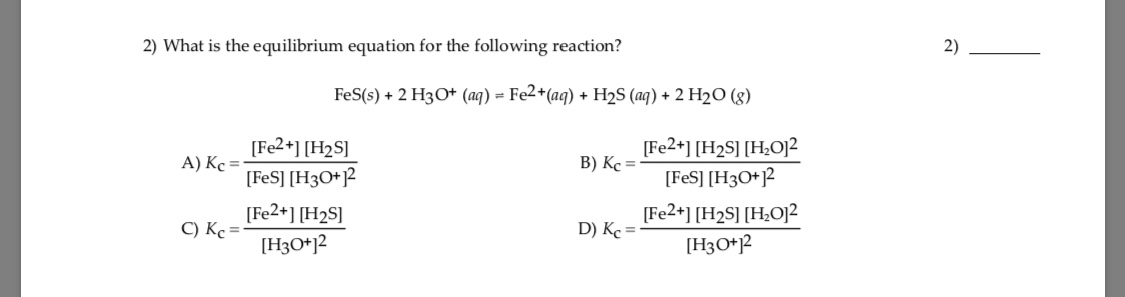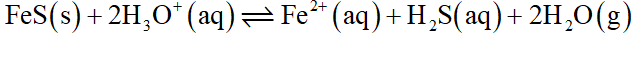# 2) What is the equilibrium equation for the following reaction?2)FeS(s) + 2 H3O* (aq) = Fe2*(aq) + H2S (aq) + 2 H2O (g)[Fe2+] [H2S]A) Kc =[FeS] [H3O+]2[Fe2+] [H2S]C) Kc =[Fe2+] [H2S] [H¿O]²B) Kc =[FeS] [H3O+?[Fe2+] [H2S] [H;O]?D) Kc =[H3O*]2[H3O*j2

Question
24 viewshelp_outlineImage Transcriptionclose2) What is the equilibrium equation for the following reaction? 2) FeS(s) + 2 H3O* (aq) = Fe2*(aq) + H2S (aq) + 2 H2O (g) [Fe2+] [H2S] A) Kc = [FeS] [H3O+]2 [Fe2+] [H2S] C) Kc = [Fe2+] [H2S] [H¿O]² B) Kc = [FeS] [H3O+? [Fe2+] [H2S] [H;O]? D) Kc = [H3O*]2 [H3O*j2 fullscreen
check_circle

Step 1

The given reaction is as follows,...

### Want to see the full answer?

See Solution

#### Want to see this answer and more?

Solutions are written by subject experts who are available 24/7. Questions are typically answered within 1 hour.*

See Solution
*Response times may vary by subject and question.
Tagged in